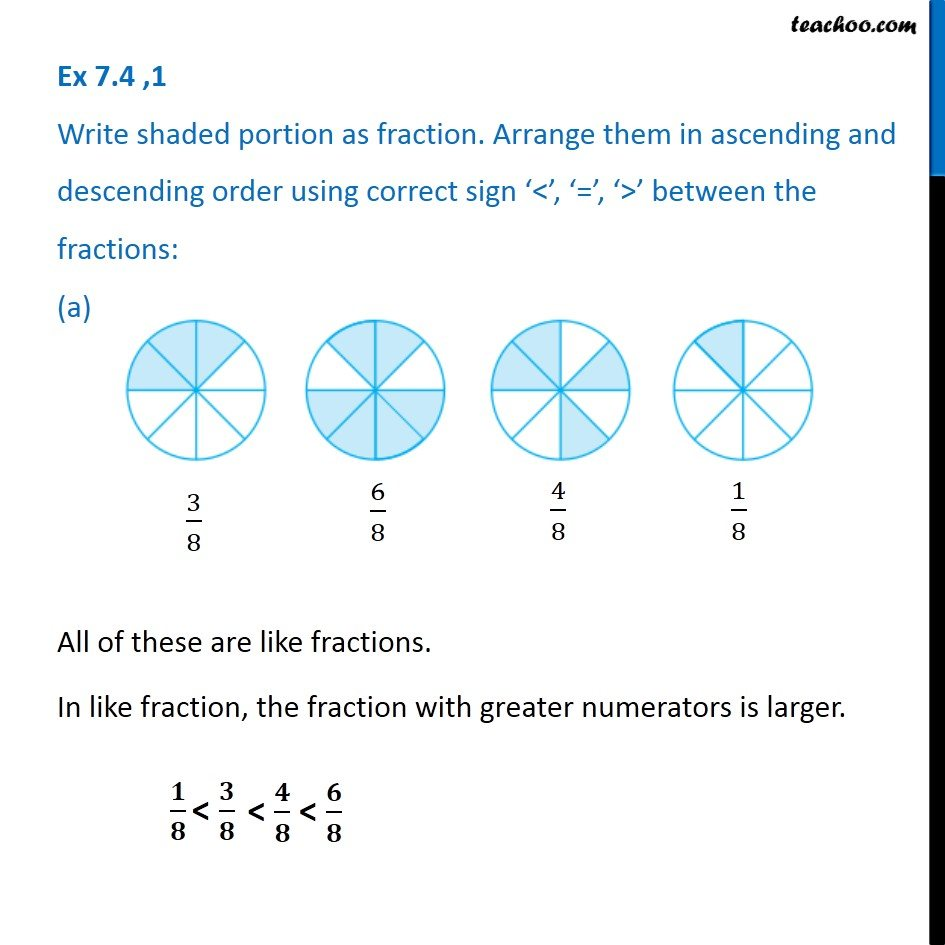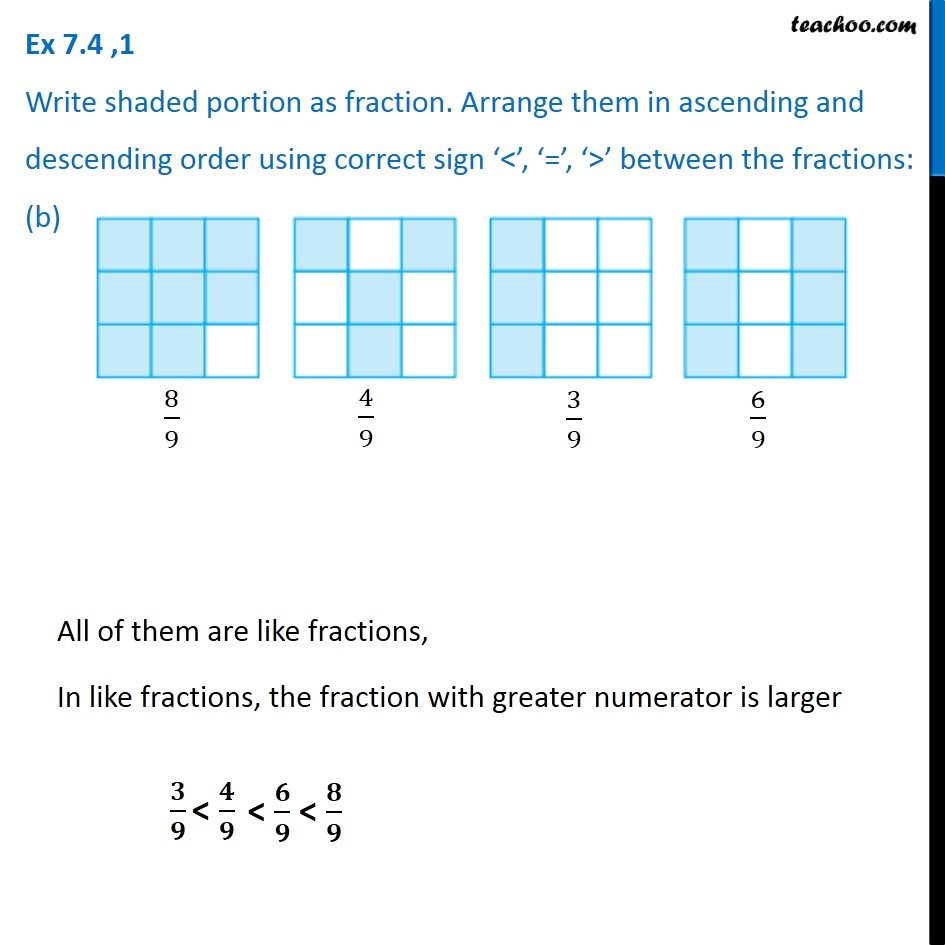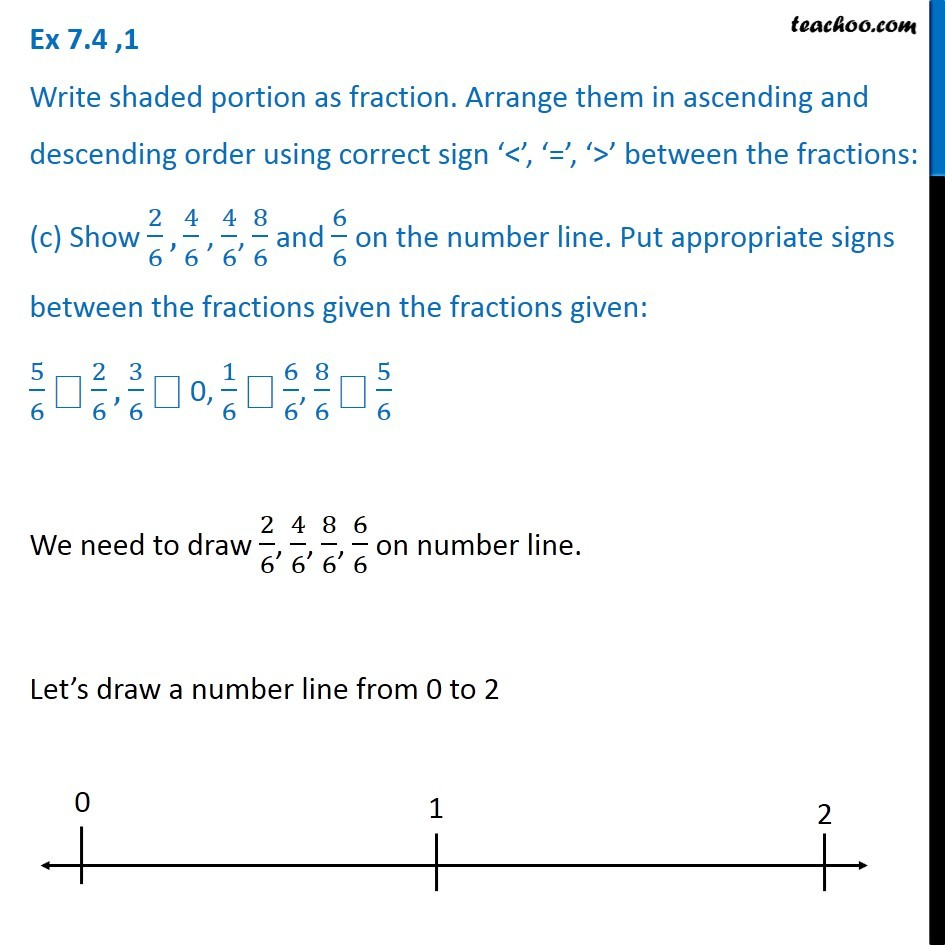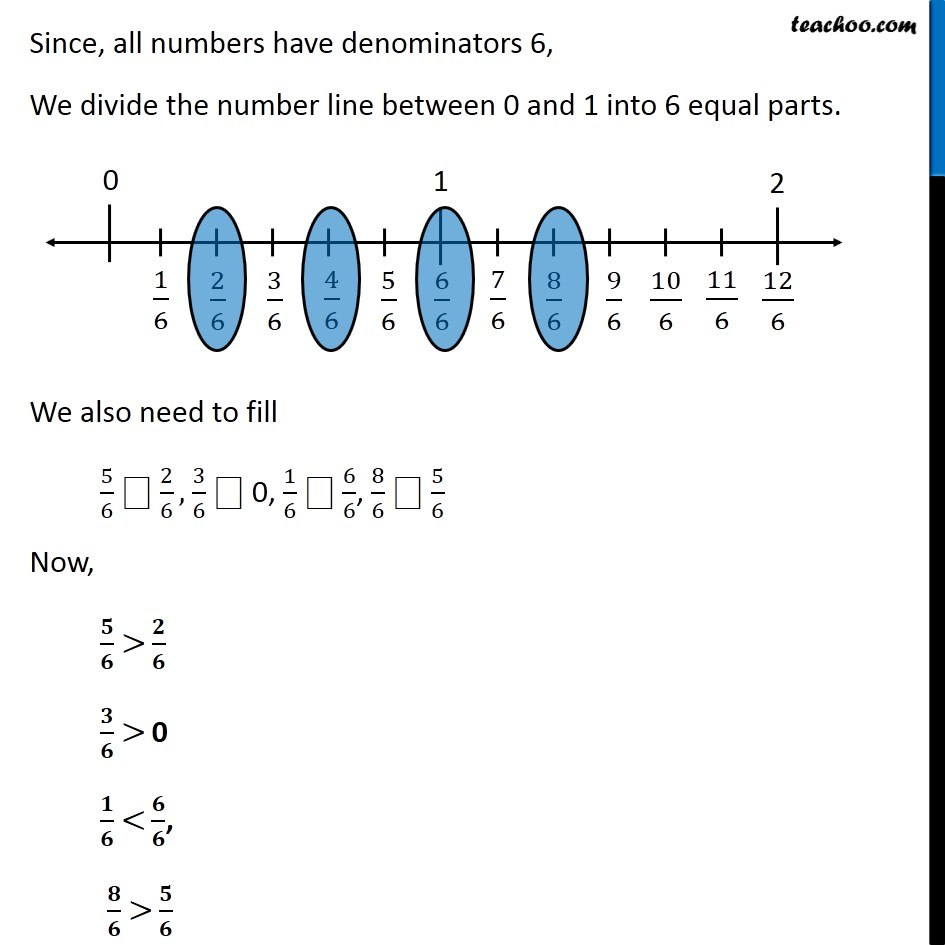1. Chapter 7 Class 6 Fractions
2. Concept wise
3. Comparing fractions

Transcript

Ex 7.4 ,1 Write shaded portion as fraction. Arrange them in ascending and descending order using correct sign ‘<’, ‘=’, ‘>’ between the fractions: (a) All of these are like fractions. In like fraction, the fraction with greater numerators is larger. Ex 7.4 ,1 Write shaded portion as fraction. Arrange them in ascending and descending order using correct sign ‘<’, ‘=’, ‘>’ between the fractions: (b) All of them are like fractions, In like fractions, the fraction with greater numerator is larger Ex 7.4 ,1 Write shaded portion as fraction. Arrange them in ascending and descending order using correct sign ‘<’, ‘=’, ‘>’ between the fractions: (c) Show 2/6, 4/6 , 4/6, 8/6 and 6/6 on the number line. Put appropriate signs between the fractions given the fractions given: 5/6 ⎕ 2/6, 3/6 ⎕ 0, 1/6 ⎕ 6/6, 8/6 ⎕ 5/6We need to draw 2/6, 4/6, 8/6, 6/6 on number line. Let’s draw a number line from 0 to 2 Since, all numbers have denominators 6, We divide the number line between 0 and 1 into 6 equal parts. We also need to fill 5/6 ⎕ 2/6, 3/6 ⎕ 0, 1/6 ⎕ 6/6, 8/6 ⎕ 5/6 Now, 𝟓/𝟔 > 𝟐/𝟔 𝟑/𝟔 > 0 𝟏/𝟔 < 𝟔/𝟔, 𝟖/𝟔 > 𝟓/𝟔

Comparing fractions